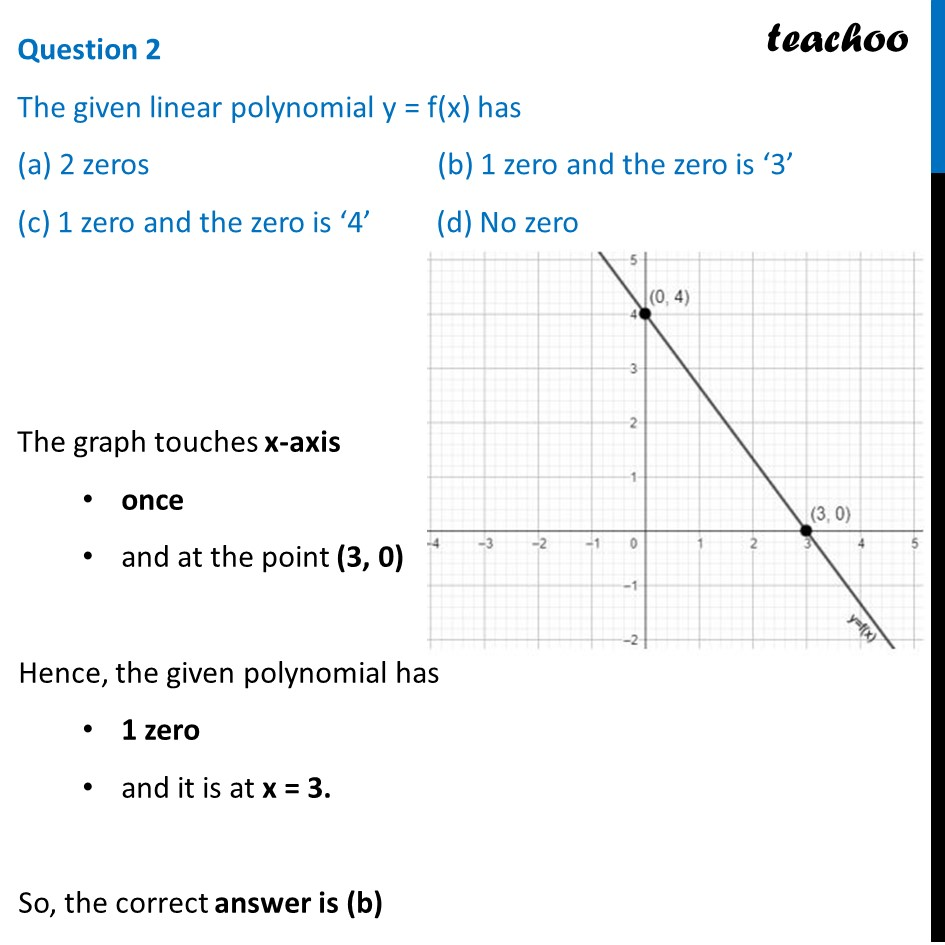CBSE Class 10 Sample Paper for 2024 Boards - Maths Standard

Class 10
Solutions of Sample Papers for Class 10 Boards

## (c) 1 zero and the zero is ‘4’             (d) No zeroLearn in your speed, with individual attention - Teachoo Maths 1-on-1 Class

### Transcript

Question 2 The given linear polynomial y = f(x) has (a) 2 zeros (b) 1 zero and the zero is ‘3’ (c) 1 zero and the zero is ‘4’ (d) No zero The graph touches x-axis once and at the point (3, 0) Hence, the given polynomial has 1 zero and it is at x = 3. So, the correct answer is (b)